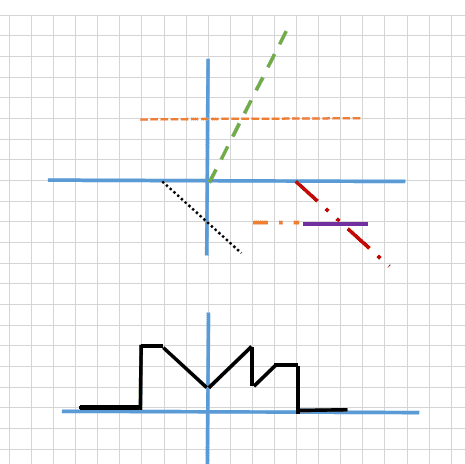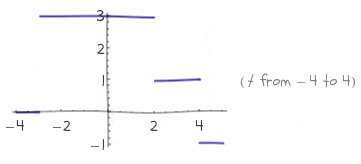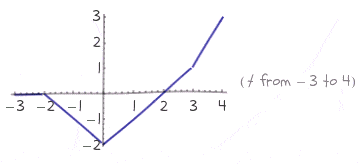# Draw heaviside and ramp function

ongxom

## Homework Statement

Plot this signal
y(t)=3u(t+3)-r(t+2)+2r(t)-2u(t-2)-r(t-3)-2u(t-4)

## The Attempt at a Solution

I tried to draw each single function, but do not know the way to plot for the whole signal like the bottom graphHomework Helper
Gold Member
Try breaking the problem down into 2 parts--a graph resulting from only the u-components, and another from only the r-components. Post what you get.

ongxom
Only for u functionOnly for r functionWhat things I have to do next to get the correct graph ?

Homework Helper
Gold Member
Your u-function looks right. Your r-function has a problem with the rightmost segment. Fix that and then just add them together. It may be easier to start with the r-function and then add the offsets from the u-function. You may even be able to do it visually if your x-axes are the same scale.

ongxom
Let me ask some question first :
Both of graph is plotted using wolframalpha, for the u-functions :
y(t)=3u(t+3)-2u(t-2)-2u(t-4)
The 3u(t+3) function is correct, but for -2u(t-4), i think it should have a line starts from (2;-2) but the graph only shows a line starts from (4;-1), and where is the line for -2u(t-2). Where does the line start from (2;1) to (4;1) come from ?

Homework Helper
Gold Member
Both of graph is plotted using wolframalpha, for the u-functions :
y(t)=3u(t+3)-2u(t-2)-2u(t-4)
Wolfram alpha would be good for checking your work, but you should first try to do the graphs manually.

The 3u(t+3) function is correct, but for -2u(t-4), i think it should have a line starts from (2;-2)
Why would you think that? The function -2u(t-4) "activates" at t=4.

but the graph only shows a line starts from (4;-1)
(4,-1) is correct.

and where is the line for -2u(t-2). Where does the line start from (2;1) to (4;1) come from ?
That would be from the third time interval (I3), below:

Realize that y(t)=3u(t+3)-2u(t-2)-2u(t-4) is composed of 4 time intervals:

I1: -∞ < t < -3 [nothing is happening because no u-function is "active" --for amplitude, think 0]
I2: -3 <= t < 2 [3u(t+3) is the only "active" --for amplitude, think 3]
I3: 2 <= t < 4, [3u(t+3) AND -2u(t-2) are both "active" --for amplitude, think 3-2]
I4: 4 <= t < ∞, [3u(t+3) AND -2u(t-2) AND -2u(t-4) are all "active" --for amplitude, think 3-2-2 ]

Last edited:
ongxom
In the definition of unit step function, the function-2u(t-4) should be
-2 if t-4>0 and 0 if t-4<0

so it should start from (4;-2), am I right ?

Homework Helper
Gold Member
Yes. Technically its: -2 if t-4 >= 0 and 0 if t-4 < 0

ongxom
Another example x(t)=2u(t)-u(t-1)-u(t+1)
For 2u(t) = 2 if t>0 and 0 if t<0, a line will start from (0;2)
For -1u(t-1) = -1 if t>1 and 0 if t<1, line starts from (+1;-1)
For -1u(t+1) = -1 if t>-1 and 0 if t<-1. line starts from (-1;-1)

I tried to plot all thing above in a graph, but does not know how to combine them into one. The correct graph have lines start from (-1;-1) to (0;-1) and (0;1) to (1;1).

I am stucking at see the way 2u(t) represent in the graph.

Last edited:
Homework Helper
Gold Member
I tried to plot all thing above in a graph, but does not know how to combine them into one.
You don't need any special tools to solve these kinds of problems. A pencil and some graph paper will do the job.

The correct graph have lines start from (-1;-1) to (0;-1) and (0;1) to (1;1).
Not really. (-∞;0) to (-1;0) to (-1;-1) to (0;-1) and (0;1) to (1;1) to (1;0) to (∞;0)

I am stucking at see the way 2u(t) represent in the graph.
2u(t) is an additive term in the composite graph. You won't "see it" in the graph as you would if it stood alone. It is responsible for the 2-unit jump from (0;-1) to (0;1).

Before jumping all over this and other examples, did you understand what I wrote in post #6? If not, what is not clear? I am trying to understand what you don't understand about the OP so please focus on post #6 for now.

ongxom
Thank you, I got the #6. Now come to the ramp function, I have tried to seperate time intervals but it seems there is nothing like the amplitude for heaviside, it doesn't work.

Homework Helper
Gold Member
What doesn't work? The time intervals for the combined ramp functions can be obtained from inspection:

yramp(t) = -r(t+2) + 2r(t+0) -r(t-3)

You know, maybe you are still trying to do too much all at once. Why not just take two of the function terms and add them together:

yramp-first-2-terms(t) = -r(t+2) + 2r(t+0)

What would that look like?

ongxom
Let me do it :
-∞<t<-2 : No signal, 0 for amplitude.
-2<t<0 : -r(t+2) is active at t=-2, -1 for amplitude.
0<t<3 : -r(t+2) and 2r(t) are both active, 2-1 for amplitude
3<t<+∞ : -r(t+2) and 2r(t) and -r(t-3) are all active, 2-1-1 for amplitude.

I have no idea what the graph would look like, the u-functions only have lines paralled with t-axis.

Last edited:
Homework Helper
Gold Member
So jumping ahead (against my advice), you are now working on yramp(t) = -r(t+2) + 2r(t+0) -r(t-3).

-∞<t<-2 : No signal, 0 for amplitude.
True.

-2<t<0 : -r(t+2) is active at t=-2, -1 for amplitude.
False. r(t) is the unit ramp function, with amplitude 0 for t<0 and a "ramping" output for t >= 0 (output starts at zero and increases linearly with a slope of 1).

-r(t+2) starts ramping at t=-2 with a slope of -1.

0<t<3 : -r(t+2) and 2r(t) are both active, 2-1 for amplitude
"amplitude" is wrong. You are thinking about the behavior of the u-function.

3<t<+∞ : -r(t+2) and 2r(t) and -r(t-3) are all active, 2-1-1 for amplitude.
Again, the "constant amplitude" concept only applies to u-functions.

I have no idea what the graph would look like, the u-functions only have lines paralled with t-axis.
You started out this post with a collection of horizontal and diagonal lines. Those diagonal lines are the the result of the r-functions. By the way, the red dash-dot-dot-dashed line is not shown correctly (it should start at t=3).